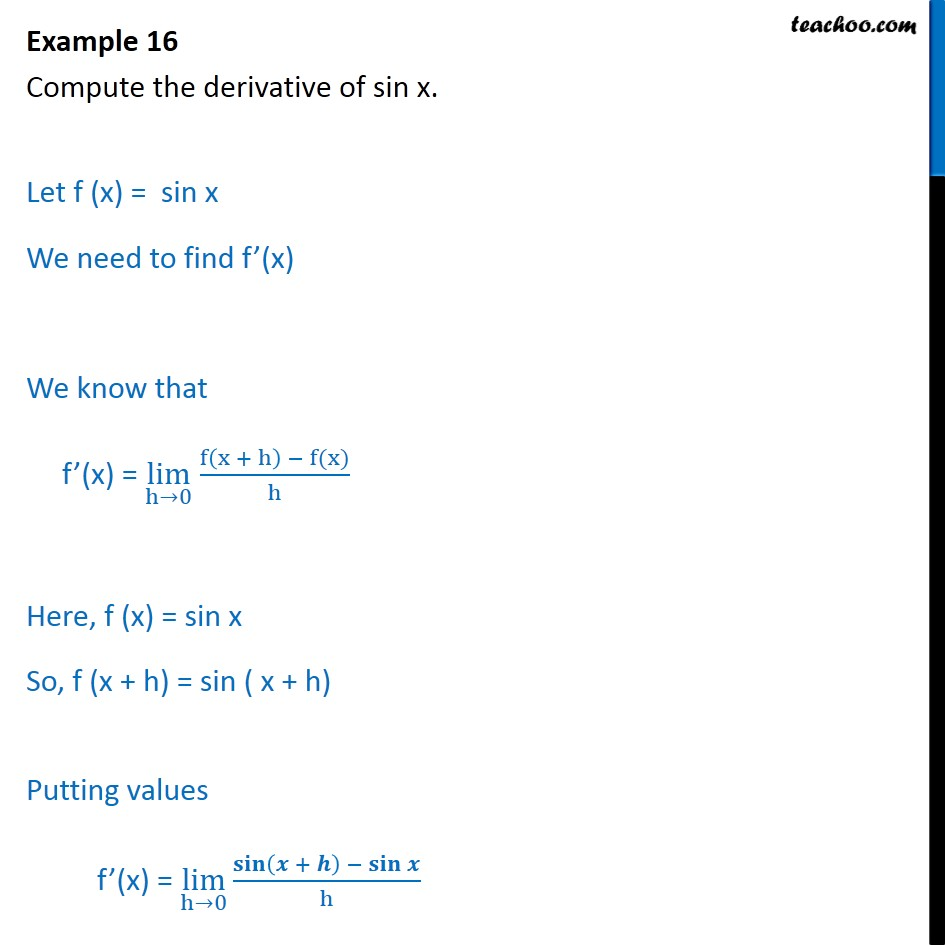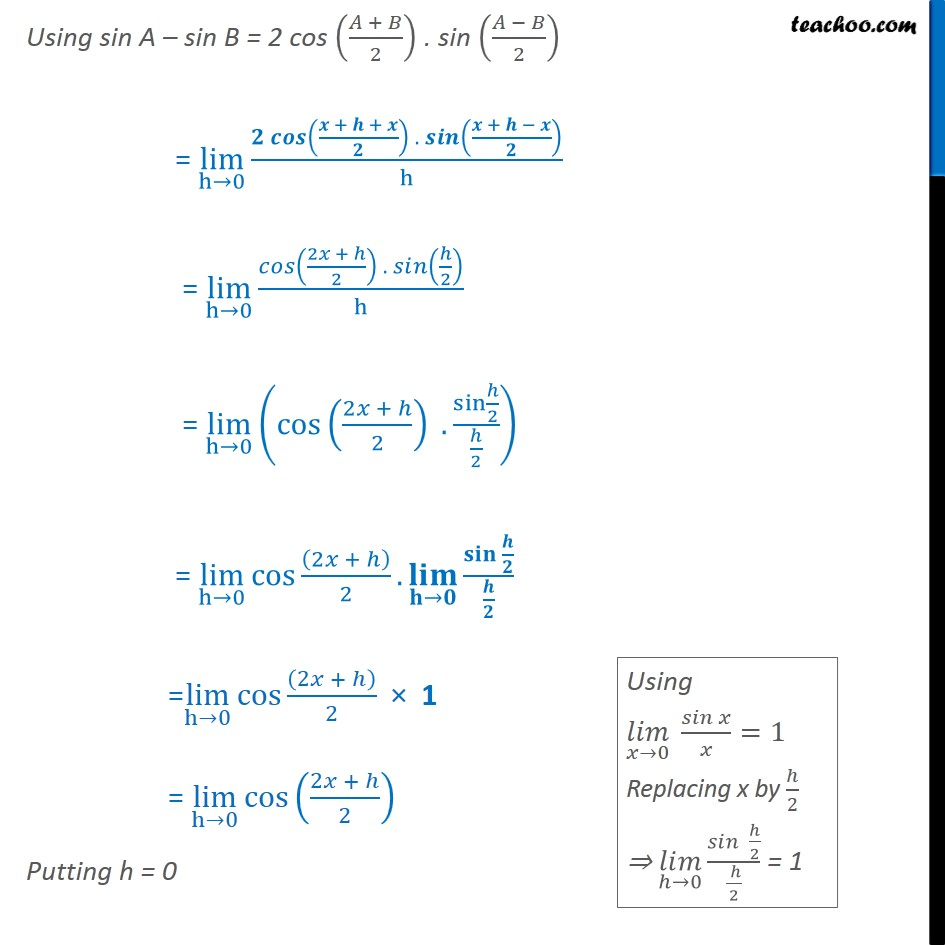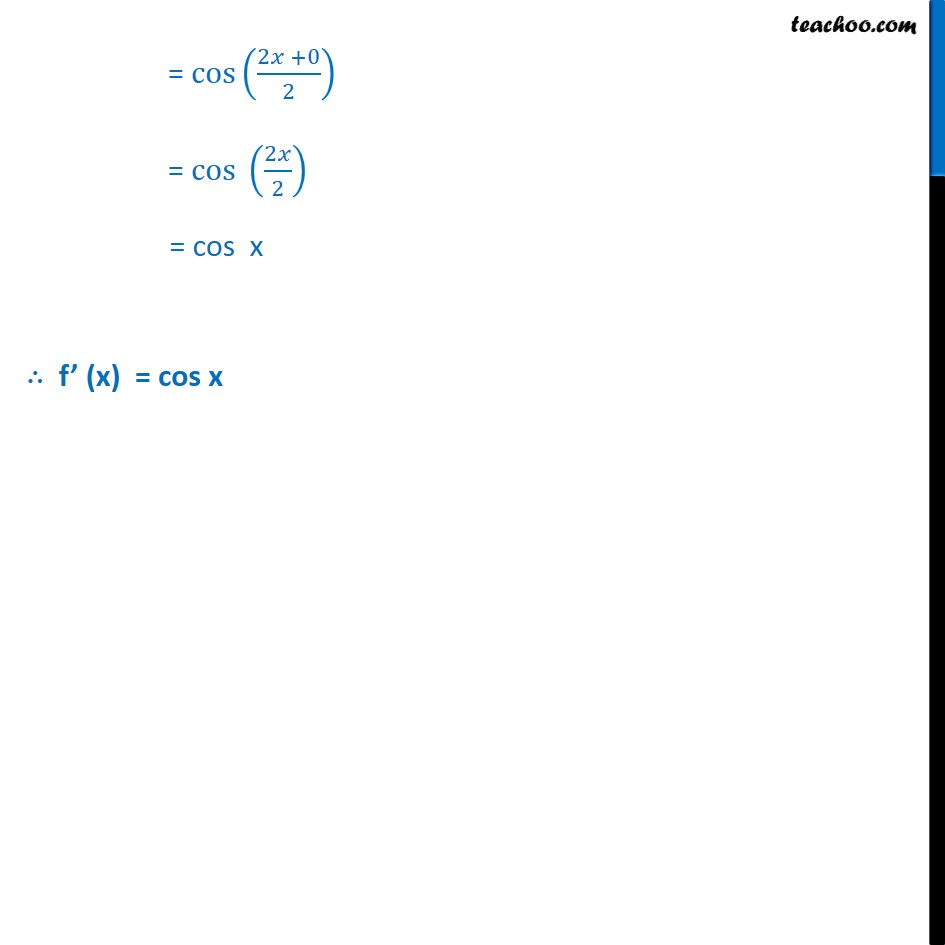Examples

Chapter 13 Class 11 Limits and Derivatives
Serial order wiseSolve all your doubts with Teachoo Black (new monthly pack available now!)

### Transcript

Example 16 Compute the derivative of sin x. Let f (x) = sin x We need to find f (x) We know that f (x) = lim h 0 f x + h f(x) h Here, f (x) = sin x So, f (x + h) = sin ( x + h) Putting values f (x) = lim h 0 + h Using sin A sin B = 2 cos + 2 . sin 2 = lim h 0 + + . + h = lim h 0 2 + 2 . 2 h = lim h 0 cos 2 + 2 . sin 2 2 = lim h 0 cos 2 + 2 . = lim h 0 cos 2 + 2 1 = lim h 0 cos 2 + 2 Putting h = 0 = cos 2 +0 2 = cos 2 2 = cos x f (x) = cos x